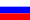# Vestnik KRAUNC. Fiz.-Mat. Nauki. 2022. vol. 39. no. 2. pp. 222–236. ISSN 2079-6641

Contents of this issue

Read Russian VersionMSC 11A63

Research Article

How to take from Pascal’s triangle an infinite series of power sums from many variables and arithmetic systems compared modulo a prime number

V. L. Shcherban’

Center for Additional Mathematical Education, 640000, Kurgan, Tomina str. 53, Russia
E-mail: sherba-q@ya.ru

In this arithmetic study, some unclaimed and unknown numerical properties of Pascal’s irregular triangles are proposed for further study. These properties made it possible to find a universal method for finding many symmetric polynomials of power sums from Pascal’s triangle tables. The same properties helped to establish two formulas for directly finding all primes. The above became available after the successful decryption of a certain group of Pascal’s triangle tables in the cryptographic subsystem. The rules of real and arithmetic operations for such tables have been found and have been unambiguously defined, therefore, implementation of the tasks on a computer is possible. Plus, there was no place for special information in combinatorial problems in the structural part of the logical material. The method of building arithmetic tables is universal and makes it possible to get their further development in the subsystem of numerical irregular triangles. Further, it has been found that only such tables can transmit arithmetic information by decryption for scientific and mathematical purposes.

Key words: recurrent numerical sequences; symmetric polynomials; prime numbers; Pascal’s triangle.

DOI: 10.26117/2079-6641-2022-39-2-222–236

Original article submitted: 05.07.2022

Revision submitted: 22.08.2022

For citation. Shcherban’ V. L. How to take from Pascal’s triangle an infinite series of power sums from many variables and arithmetic systems compared modulo a prime number. Vestnik KRAUNC. Fiz.-mat. nauki. 2022, 39: 2, 222–236. DOI: 10.26117/2079-6641-2022-39-2-222–236

Competing interests. The author declares that there are no conflicts of interest with respect to authorship and publication.
Contribution and responsibility. The author contributed to the writing of the article and is solely responsible for submitting the final version of the article to the press. The final version of the manuscript was approved by the author.

References

1. Voronin S. M. Prostyye chisla [Simple numbers], Moscow, Znaniye, 1978, p. 95 (In Russian).
2. Prasolov V. V. Mnogochleny [Polynomials], Moscow, MTsNMO, 2001, p. 336 (In Russian).
3. Vinberg E. B. Algebra mnogochlenov [Algebra of polynomials], Moscow, Prosveshcheniye, 1980, p. 176 (In Russian).
4. Markushevich A. I. Vozvratnyye posledovatel’nosti [Return sequences], Moscow, Nauka, 1983, p. 48 (In Russian).
5. Uspenskiy V. A. Treugol’nik Paskalya [Pascal’s triangle], Moscow, Nauka, 1979, p. 46 (In Russian).
6. Bogatyrev R. R. Zolotoy treugol’nik, Mir PK, 2001, no. 6, pp. 52–60 (In Russian).
7. Vorob’yev N. N. Chisla Fibonachchi [Fibonacci numbers], Moscow, Nauka, 1992, p. 189 (In Russian).
8. Gel’fond A. O. Resheniye uravneniy v tselykh chislakh [Solution of equations in integers], Moscow, Nauka, 1978, p. 62 (In Russian).
9. Boltyanskiy V. G., Boltyanskiy N.Ya. Simmetriya v algebre [Symmetry in algebra], Moscow, MTsNMO, 2002, p. 240 (In Russian).
10. Sposob dokazatel’stva dlya matematiki, gde sushchestvuyet mnogo suzhdeniy, kotoryye ne mogut byt’ dokazany po-drugomu [Electronic resource]. Access mode: https://ru.wikipedia.org/wiki/Dokazatel’stvo_ot_protivnogo (date of access: 07.02.2021) (In Russian).
11. Bykov V.I., Kytmanov A.M., Lazman M.Z. Metody isklyucheniya v komp’yuternoy algebre mnogochlenov [Elimination methods in computer algebra of polynomials], Novosibirsk: Nauka. Sib. otd-niye, 1991, p. 231 (In Russian).
12. Vygodskiy M.Ya. Spravochnik po elementarnoy matematike [Handbook of elementary mathematics], Moscow, Gostekhizdat, 1954, p. 412 (In Russian).
13. Shcherban’ V. L. Pochemu okruzhayushcheye nas prostranstvo imenno trekhmerno, Vestnik Baltiyskogo federal’nogo universiteta im. I. Kanta. Ser. Fiziko-matematicheskiye i tekhnicheskiye nauki, 2020, no. 1., pp. 97–112, (In Russian).
14. Ayoub R. An Introduction to the Analitic Theory of Numbers. American mathematical society providence, Rhode Island. 1963. p. 377.
15. Itogi nauki i tekhniki. Seriya «Sovremennaya matematika i yeye prilozheniya. Tematicheskiye obzory». Math-net.ru arkhiv [Elektronnyy resurs]. Access mode: http://www.mathnet.ru/php/journal.phtml?jrnid=into&wshow=details&option_lang=rus (date of access: 07. 02. 2021) (In Russian). Scherban’

Scherban’ V. L. – Head of the educational Department, Center for Additional Mathematical Education, Kurgan, Russia, ORCID 0000-0002-5631-9681.